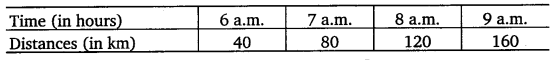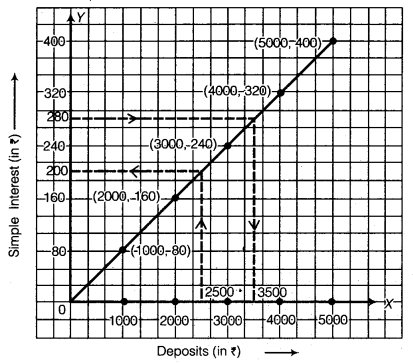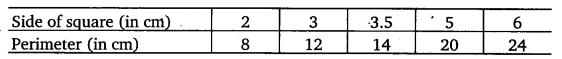# Class 8 Maths NCERT Solutions for Chapter – 15 Introduction to Graphs Ex – 15.3

## Introduction to Graphs

Question 1.
Draw the graphs for the following tables of values, with suitable scales on the axes.
(a)
Cost of apples

(b)
Distance travelled by a car(i) How much distance did the car cover during the period 7.30 a.m. to 8 a.m.?
(ii) What was the time when the car had covered a distance of 100 km since it’s start? Interest on deposits for a year

(c) Interest on deposits for a year.(i) Does the graph pass through the origin?
(ii) Use the graph to find the interest on ( 2500 for a year.
(iii) To get an interest of? 280 per year, how much money should be deposited?

Solution:
(a) In order to represent the given data graphically, we represent ‘Number of apples, on the x-axis and ‘Cost of apples (in ₹)’ on the y-axis. The ordered pairs (1, 5), (2, 10), (3, 15), (4, 20) and (5, 25) are plotted as points and are joined by the line segments as shown in the figure. This gives the required graph.

(b) In order to draw time-distance graph, we represent the time (in hours) on x-axis and the distances (in km) on y-axis.
Scale : 2 units = 1 hour on x-axis and 1 unit = 10 km on y-axis.
Plot the points (6, 40), (7, 80), (8, 120) and (9, 160).
Join them by the line segments to obtain the required graph given below :

(i)
Clearly, from the graph E” distance covered by the s car during 7.30 a.m. to g 8.00 a.m. is 20 km. §
(∵ 120 -100 =20 km)
(ii) The time when the car had covered 100 km since it’s start is 7.30 a.m.

(c) In order to draw principal-simple interest graph, we represent deposits (in X) on x-axis and the simple interest (in ₹) on y-axis.
Scale : 1 unit = ₹ 1000 on x-axis.
1 unit = ₹ 80 on y-axis.
Plot the points (1000, 80), (2000, 160), (3000, 240), (4000, 320) and (5000, 400).Join these points to obtain the required graph.
Yes, the graph passes through origin.
(ii) Corresponding to ₹ 2500 on the x-axis, we get the interest to be ₹ 200 on the y-axis.
(iii) Corresponding to ₹ 280 per year on the y-axis, we get the sum to be ₹ 3500 on the x-axis.

Question 2.
Draw a graph for the following :
(i)Is it a linear graph?
(ii)Is it a linear graph?

Solution:
(i) In order to represent the given data graphically, we represent the side of square (in cm) on the x-axis and the perimeter (in cm) on the y-axis. Scale : 1 units = 1 cm on x-axis and y-axis.
Plot the points (2, 8), (3, 12), (3.5, 14), (5, 20) and (6, 24). Join these points to obtain the required graph.
It is a linear graph.

(ii)
In order to represent the given data graphically, we represent the side of square (in cm) on the x-axis and the area (in cm2) on the y-axis. Scale : 1 unit = 1 cm on x-axis and 1 unit = 1 cm2 on y-axis.
Plot the points (2, 4), (3, 9), (4, 16), (5, 25) and (6, 36). Join these points to obtain the required graph.It is not linear.

### Take Your IIT JEE Coaching to Next Level with Examtube

• Mentoring & Teaching by IITians
• Regular Testing & Analysis
• Preparation for Various Engineering Entrance Exams
• Support for School/Board Exams